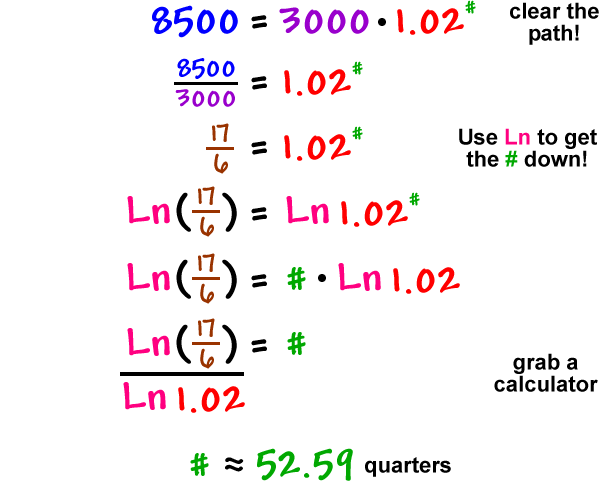Now that we know how to solve exponential equations, let's go back to money and populations and solve some harder problems.

How long will it take \$3000 to grow to \$8500 if it is invested in an account that earns 8% compounded quarterly?

Set it up:

initial amount = 3000

final amount = 8500

split factor = 1.02

( remember that, each quarter, \$1.00 splits into \$1.02! )

We are looking for time...

We'll need to get the number of splits, #, then adjust it to years.That's about 13.15 years.  (I divided by 4!)